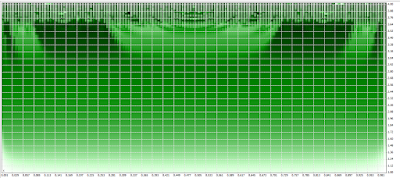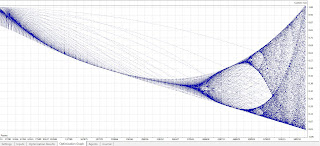## Chaos kernel function using the logistic map?

### Chaos kernel function using the logistic map?

Last updated 835 days ago by JohnLast

I made some experiments with different kernels and I would like to share among friends some ideas.

Well it is about to use a function expressing chaotic behaviour itself as a kernel.

The simplest thing was to look at the most simple logistic map.

Xn+1 = r Xn (1 - Xn)

That is the equation of the logistic map. What we can do with that.

What is interesting for us is the parameter r. If we vary the parameter we will have different behaviour (sensitivity of the initial conditions). I keep it short here but we can get some very interesting stretching-and-folding structures , please look at the Wikipedia article.This is a 3d plot of the logistic map (with just iteration ) for x varying between 0.001 and 1. and r from 1 to 2.

And this is with just one cycle. It is necessary to use parameters from 0 to 1 if more iterations are going to be used.

And this can be used as a kernel for some specific trading strategies. I have in mind some strategies like EURCHF strategy.

However we can go further.

So the idea as as follows:

Given the logistic map equation:

Xn+1 = r Xn (1 - Xn)

we can vary three parameters and to use this as a kernel:

1. We can vary x (from 0 to 1 with step 0.01, or 0.001 for example): this is giving us the initial conditions

2. We can vary the r parameter (from 1 to with step 0.01, or 0.001 for example) this is the parameter governing the chaos. r approximately 3.57 is the onset of chaos, so we can limit from 1 to 3.

3. And of course finally we can vary the limitmap parameter governing the number of iterations. This is the third parameter for the difference equation. Here I need some help because the code I made is missing to plot the values of first two iterations and it is beginning to plot from the third.

So We can call this chaos kernel, in fact I do not know even if that has a name. I did not find in the available litterature anything of this kind. So it is possible that this appears on this site for the first time. I know crazy it is.

The two MAIN ideas ARE:

- TO USE RECURRENT DIFFERENCE EQUATION GENERATING COMPLEX BEHAVIOR with GENETIC OPTIMIZER

- TO USE SOME PORTION OF CHAOS OF THE KERNEL ITSELF

If that is true with just one kernel we have something extremely powerfull. And even more, there are many other maps waiting to be exploited as kernels.

I am using here the logistic map because it is something extremely simple but generating extremely complex mathematical behavior.

Using chaos kernel to harness the chaos itself ;). Why not after all?

Below you can see the plot under MT5 of the logistic map.

This is the MT5 code (I miss the first two iterations any help would be welcome).

Here is the code of the logistic kernel (chaos kernel, in fact it does not have a name yet) you can implement in your trading strategies:

double chaoskernel()

{

//---

double w, j;

w=(r*x*(1-x));

for(j=0;j<=limitmap;j++)

{

w=(r*w*(1-w));

}

double p1 = iCustom(TimeFrame,Symbol(),"PFE",x5,true,5,0,0);// This is a NORMALIZED input

return(w* p1);// We multiply the input value by the weight

}